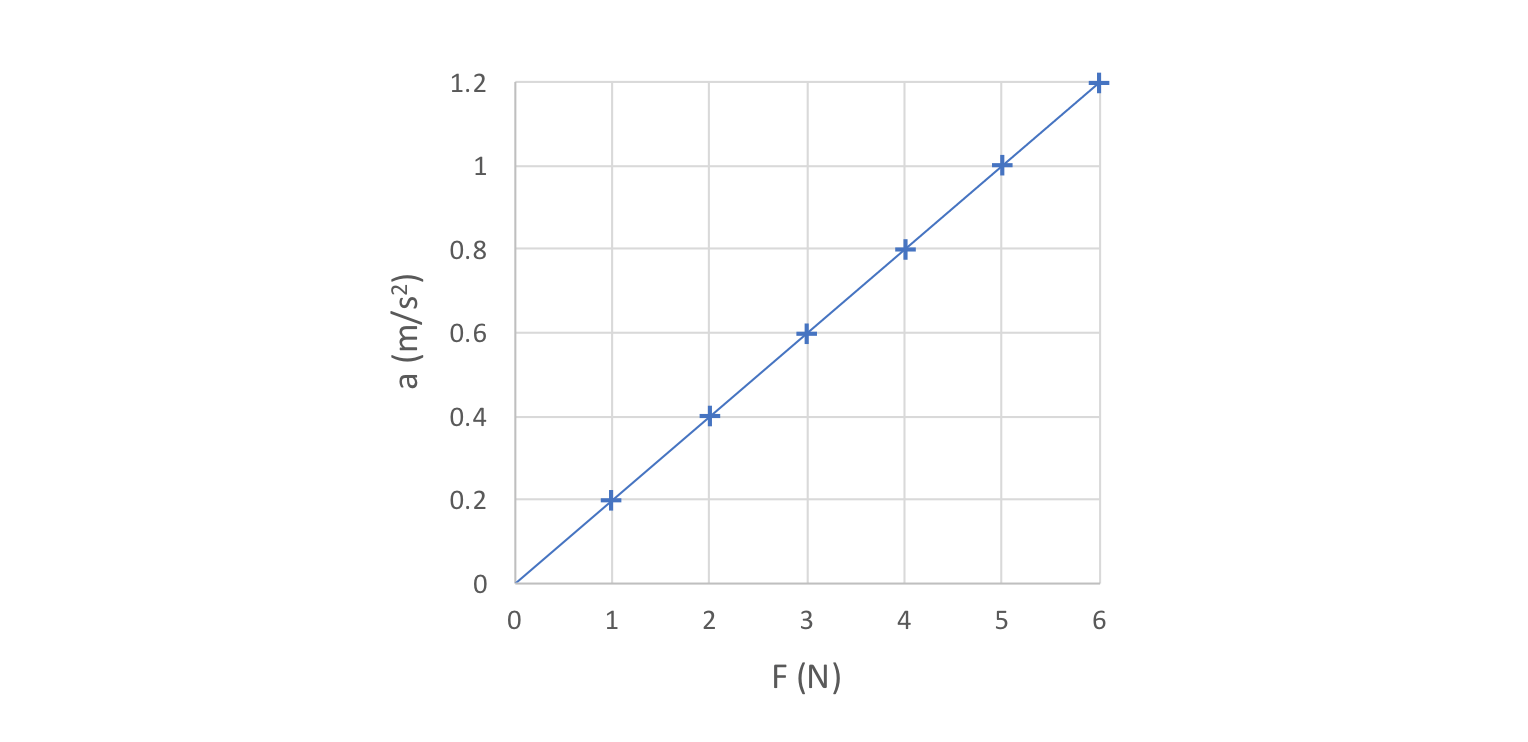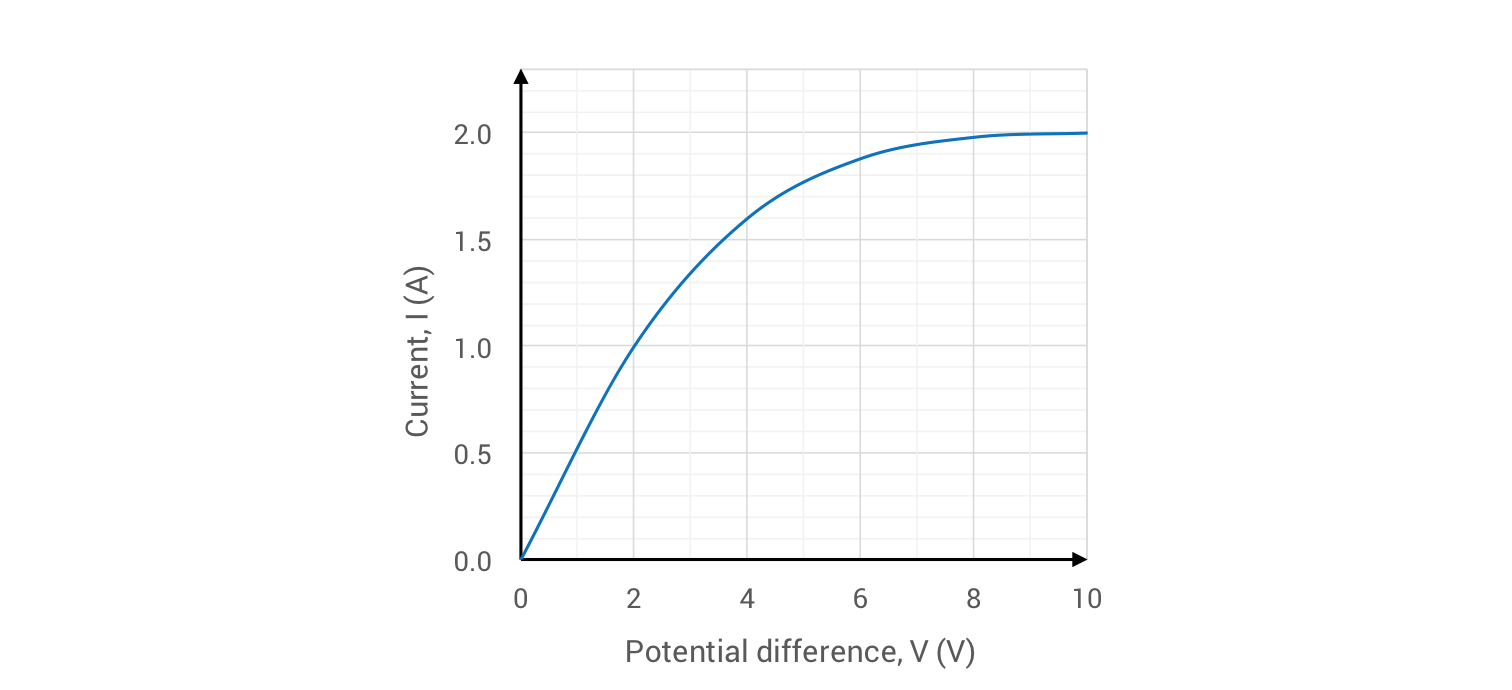Physics 31/10/2018

# Mathematical relationships in GCSE Physics

By KEVIN QUINN

Sometimes students treat an equation like a black box. You plug in the numbers and – hey presto! – out comes the answer. But if you’re going for a top grade, you need to think carefully about the meaning of the equation and how its variables are related to one another. In this blog, we explain proportionality and constants, as well as linear and nonlinear relationships.

As you can see from our blog Equations in GCSE Physics, there are a lot of equations in the new 9-1 GCSE Physics course. The best way of remembering them all is to understand how they work. Here, we take a closer look at proportionality and constants, as well as linear and nonlinear relationships.

## Direct proportionality

Let’s get stuck right into an example. Here goes.

EXAMPLE.  Erica investigates how the acceleration of a trolley is affected by the resultant force applied to it, and obtains the following results.She then states that the acceleration of the trolley is directly proportional to the resultant force applied to it. Is she correct? Explain your answer.

SOLUTION.  If two variables are directly proportional to one another it means that, as one doubles in size, then so does the other; if one trebles, then so does the other, or if one halves, then so does… well, you get the idea. We say that the proportional change in one variable is equal to the proportional change in the other.

In this example, the statement that the acceleration is proportional to the resultant force can be written mathematically as:

## a ∝ F

Take a look at the data above.  Does a double as F doubles?  Well, in the first pair of readings, when F = 1 N, a = 0.2 m/s2. In the second pair of readings, when F has doubled to 2 N, a has also doubled to 0.4 m/s2. This pattern continues for the rest of the data (compare F = 2 with F = 4, or F =3 with F = 6).

To get the marks in this question in an exam, you would answer “yes”, and then compare any two pairs of values as we’ve done above.

## Proportionality to equality

The proportionality relation between acceleration and resultant force (a ∝ F) is really useful, as it helps us see quickly how changing F affects a. But it isn’t an equation. Why not? Because it doesn’t contain an equals sign, of course!

To turn any proportionality relation into an equation, we have to add a constant of proportionality.  In maths, this constant is normally given the symbol k, which leads us to the following equation which relates a to F:

## a = k F

If you’ve already watched the video on Newton’s laws of motion then you might have spotted that, in this equation, the value of k is equal to 1/m (where m is the mass of the trolley). Multiplying both sides of this equation by m leads us to the usual mathematical version of Newton’s second law (F = m a).

Another way to prove that a ∝ F is to calculate the value of k for each pair of values. This is done by dividing each value of a by the corresponding value of F. By doing this, we end up with an additional row in our table:Calculating the value of k for several pairs of values is another way to check whether a and F are proportional to one another in this dataset. If the values which we calculate for this constant of proportionality (k) are all equal to one another, then we can say with confidence that a is indeed proportional to F.

## See the word linear? Think ‘line’

The relationship between a and F in the previous example is linear.  When you see the word linear in physics, think ‘line’. A graph of two variables which are directly proportional to one another will always be a straight line through the origin.

In this case, we can see that a plot of a against F is indeed a straight line through the origin, and that a doubles when F doubles (and so on). This is further confirmation of the fact that a ∝ F.## Curveball

If the variables in physics were always directly proportional to one another, and we lived in a world of straight lines, then we’d finish this blog right here and pat ourselves on the back for another job well done. But not so fast! Let’s look at another example.

EXAMPLE.  Howard investigates how the current (I) through a filament bulb changes as the potential difference (V) across it is increased, and obtains the below results.Use this data to show that the current is not directly proportional to the potential difference across the bulb.

SOLUTION.  If you follow the steps taken in the solution to the previous example, you’ll quickly be able to prove that I is not proportional to V for this dataset. For example, when V increases from 2 to 4, I only increases from 1.00 to 1.60. In other words when V doubles, I increases by a factor of less than 2. That’s all you need to say to answer this question in an exam.

Looking more closely, we can see that if we divide each I value by the corresponding V value (1.00 ÷ 2, 1.60 ÷ 4, etc.) the calculated values aren’t equal to one another. That’s further proof that I and V are not proportional in this case. Finally, if you want to be triply sure that I and V are not directly proportional to one another here, we can plot a graph of I against V.This relationship is described as being nonlinear, as the above graph is not a straight line. In this case current is not directly proportional to the potential difference across the bulb.

Kevin Quinn